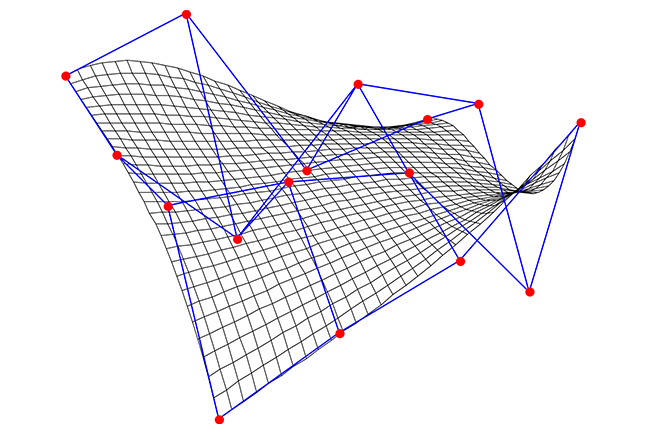2.25

## UNSW SydneyBézier surface example

# Parametric forms for lines and vectors

In many situations, it is useful to have an alternative way of describing a curve besides having an equation for it in the $\normalsize{x-y}$ plane. A parametric form for a line occurs when we consider a particle moving along it in a way that depends on a parameter $\normalsize{t}$, which might be thought of as time. Thus both $\normalsize{x}$ and $\normalsize{y}$ become functions of $\normalsize{t}$. The simplest parameterisation are linear ones.

In this step we

• look at parameterisations of the simplest curves: lines

• show how to go from parametric equations to Cartesian equations

• introduce vectors.

## Parameterisations of a line

A simple example of a parameterisation is a linear parameterisation:

As $\normalsize{t}$ varies, the point $\normalsize{p(t)}$ moves along a line. When $\normalsize{t=0}$, $\normalsize{p(0)=[1,-3]}$, when $\normalsize{t=1}$, $\normalsize{p(1)=[2,-1]}$, and when $\normalsize{t=2}$, $\normalsize{p(2)=[3,1]}$. Clearly also fractional values of $\normalsize{t}$ are allowed.How do we go from a parametric form for a line to one of the more usual Cartesian forms involving $\normalsize{x}$ and $\normalsize{y}$? We eliminate the parameter $\normalsize{t}$. For example for the parametric equation $\normalsize{p(t)=[t+1, 2t-3]}$ we have

so that $\normalsize{y=2(x-1)-3}$ or $\normalsize{y=2x-5}$. Please check that this is the same line – with a different equation.Q1 (E): Find a Cartesian equation for the parametric line ${\normalsize p(s) = [2s+1, 4s-3]}$.

## How to go from a Cartesian equation to a parametric form?

On the other hand, how can we go from a Cartesian equation of a line, say $\normalsize{y=3x+2}$ to a parametric form? It is important to realise that although the Cartesian form is more or less unique, this is not at all the case for a parametric form.

One way is to introduce a parameter in a simple way, say by setting $\normalsize{x=t}$. Then the original equation gives $\normalsize{y=3x+2=3t+2}$. Putting these together gives the parameterisation $\normalsize{p(t)=[t,3t+2]}$.

Q2 (E): Find another parameterisation of $\normalsize y=3x+2$ by setting $\normalsize x=3t$.

## Using vectors to parameterise lines

The rest of this step is about vectors, for those who are familiar with them. A vector is a directed line segment, or geometrically a difference between two points in the plane. We will denote vectors by round brackets, such as $\normalsize{v=(1,4)}$. This represents a relative displacement of $\normalsize{1}$ to the right in the $\normalsize x$-direction and $\normalsize{4}$ up in the $\normalsize y$-direction. We agree that vectors are not fixed in place, as they are only determined in a relative sense.

Suppose that we consider the line through the point $\normalsize{[3,2]}$ which goes in the direction $\normalsize{v=(1,4)}$. This line can be expressed as

which is now in parametric form with parameter $\normalsize{t}$.

What is the Cartesian equation of this line? We may eliminate $\normalsize{t}$ from the equations $\normalsize{x=3+t}$, $\normalsize{y=2+4t}$ to get $\normalsize{y=2+4(x-3)=4x-10}$ so the equation is $\normalsize{y=4x-10}$.A1. This is also $\normalsize{y=2x-5}$.
A2. Another parameterisation is $p(t) = [3t, 9t+2]$.